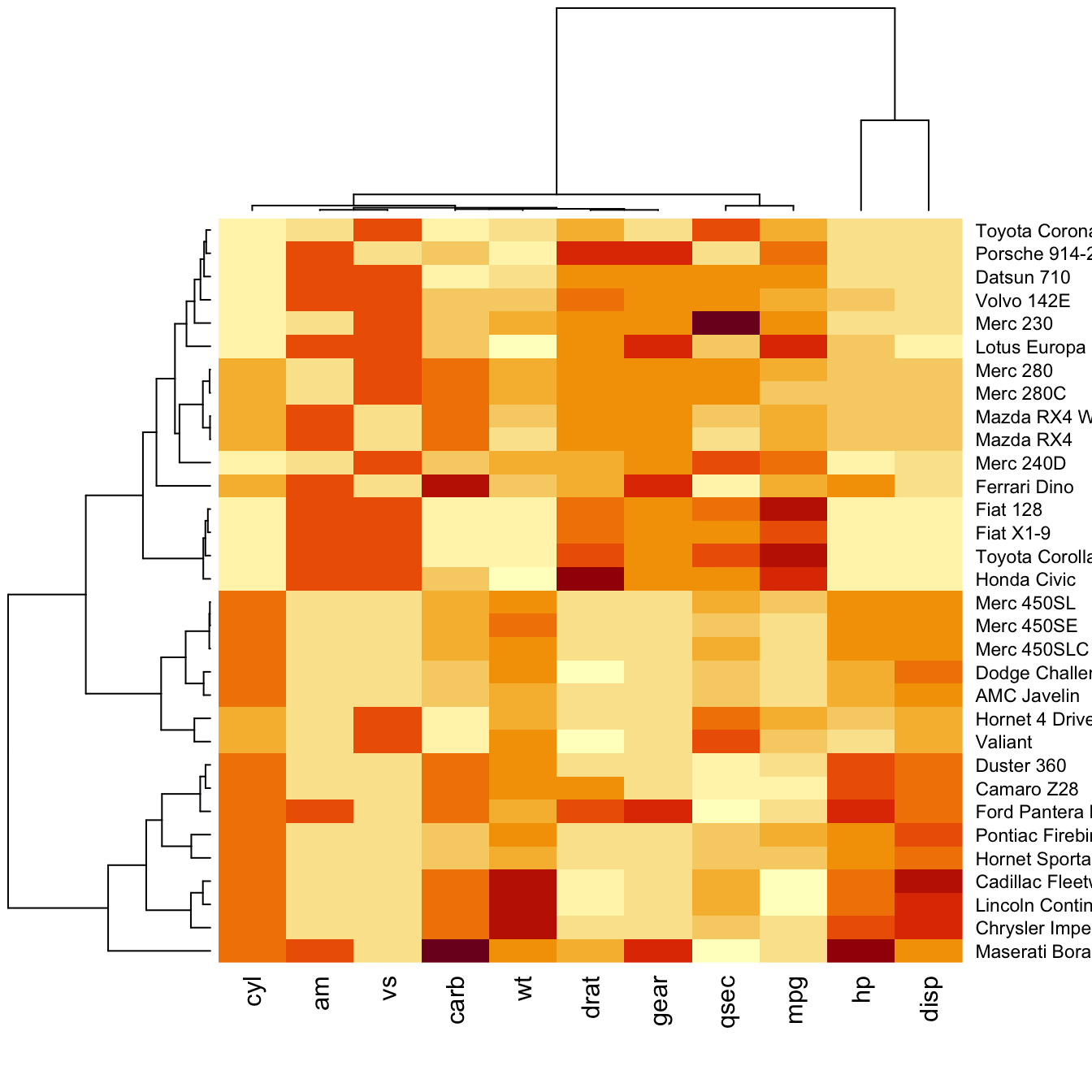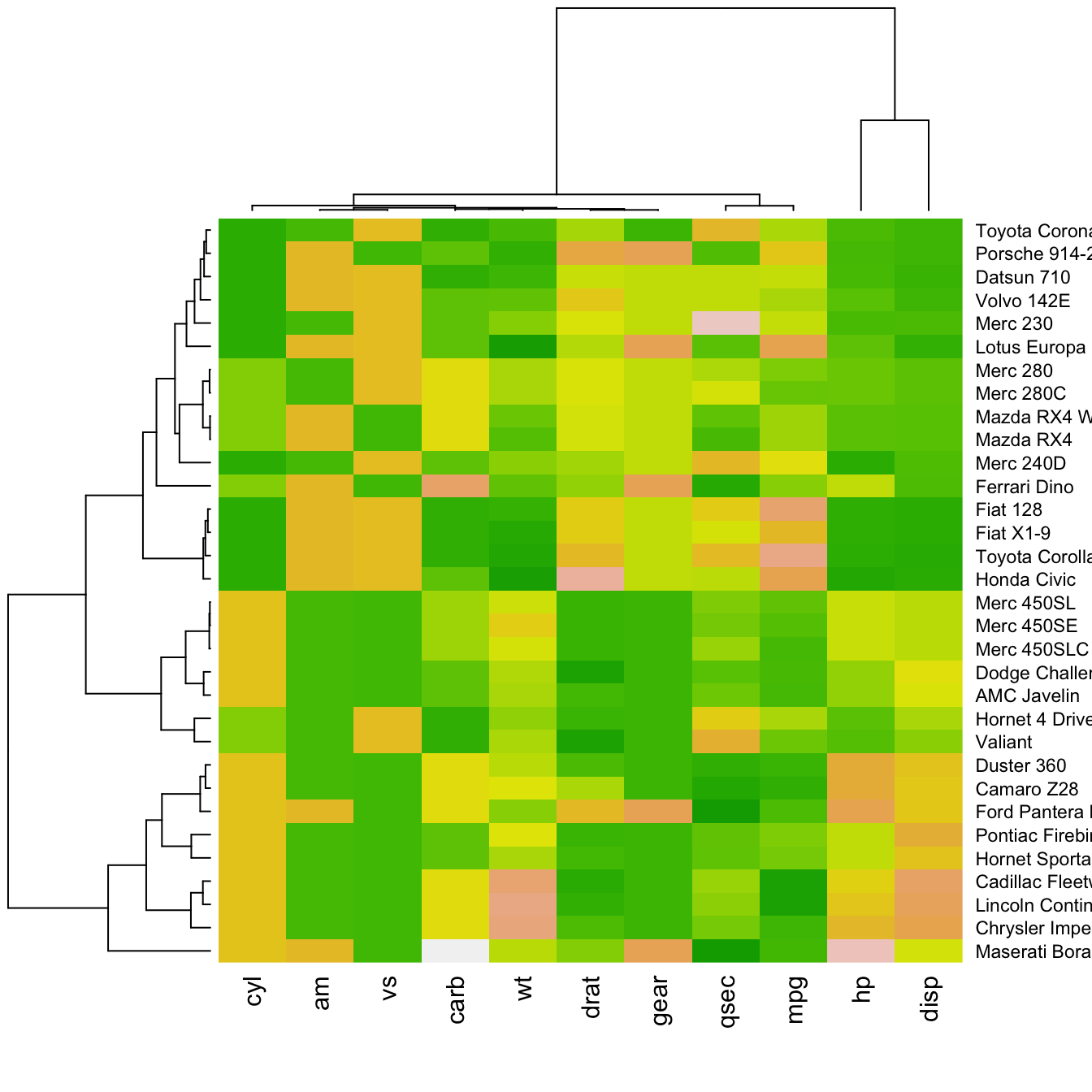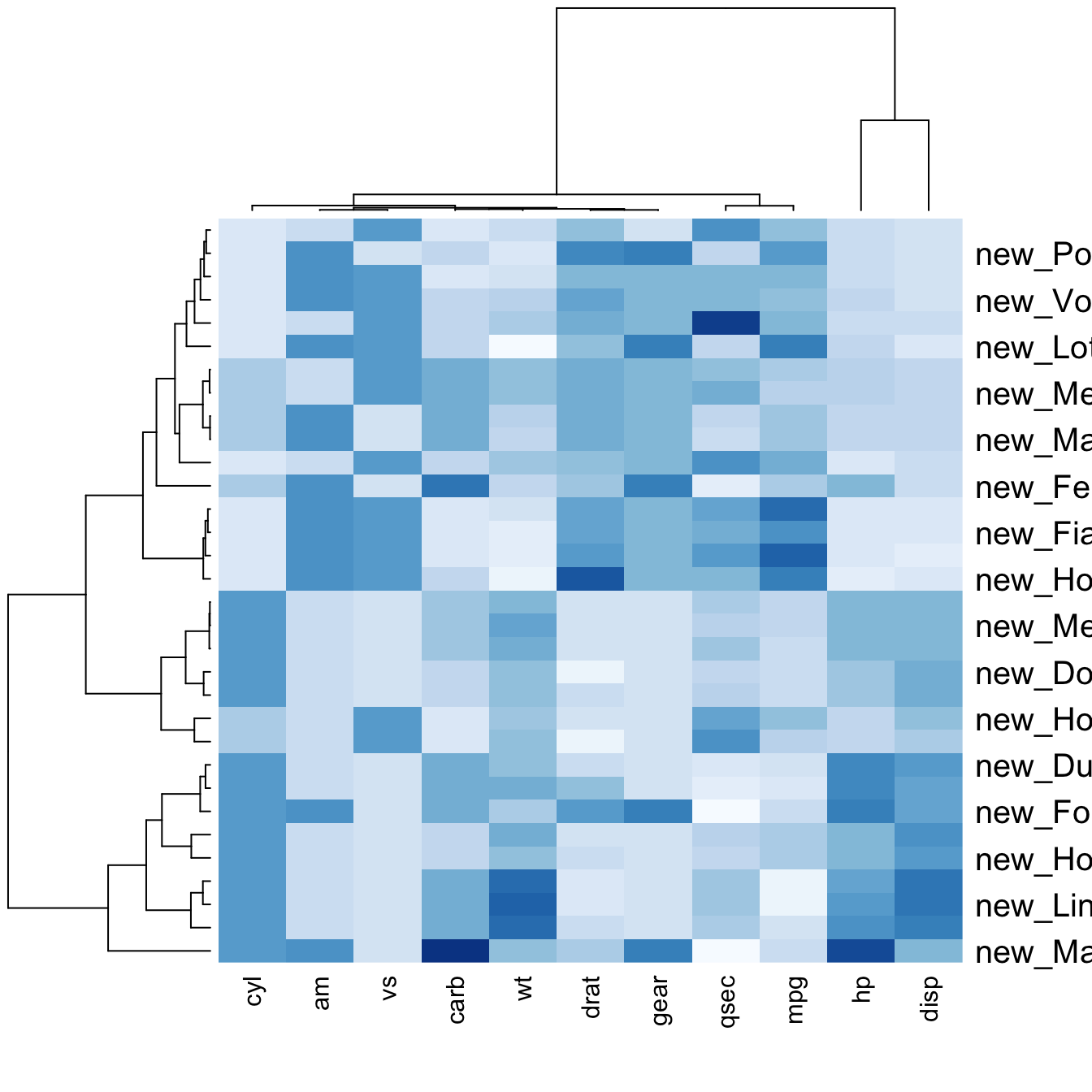# Building heatmap with R

A complete explanation on how to build heatmaps with base R: how to use the `heatmap()` function, how to custom appearance, how to normalize data and more.

# Most basic Heatmap

How to do it: below is the most basic heatmap you can build in base R, using the `heatmap()` function with no parameters. Note that it takes as input a matrix. If you have a data frame, you can convert it to a matrix with `as.matrix()`, but you need numeric variables only.

How to read it: each column is a variable. Each observation is a row. Each square is a value, the closer to yellow the higher. You can transpose the matrix with `t(data)` to swap X and Y axis.

Note: as you can see this heatmap is not very insightful: all the variation is absorbed by the `hp` and `disp` variables that have very high values compared to the others. We need to normalize the data, as explained in the next section.``````# The mtcars dataset:
data <- as.matrix(mtcars)

# Default Heatmap
heatmap(data)``````

# Normalization

Normalizing the matrix is done using the `scale` argument of the `heatmap()` function. It can be applied to `row` or to `column`. Here the `column` option is chosen, since we need to absorb the variation between column.``````# Use 'scale' to normalize
heatmap(data, scale="column")``````

# Dendrogram and Reordering

You may have noticed that order of both rows and columns is different compare to the native `mtcar` matrix. This is because `heatmap()` reorders both variables and observations using a clustering algorithm: it computes the distance between each pair of rows and columns and try to order them by similarity.

Moreover, the corresponding `dendrograms` are provided beside the heatmap. We can avoid it and just visualize the raw matrix: use the `Rowv` and `Colv` arguments as follow.``````# No dendrogram nor reordering for neither column or row
heatmap(data, Colv = NA, Rowv = NA, scale="column")``````

# Color paletteThere are several ways to custom the color palette:

• use the native palettes of R: `terrain.color()`, `rainbow()`, `heat.colors()`, `topo.colors()` or `cm.colors()`
• use the palettes proposed by `RColorBrewer`. See list of available palettes here.
``````# 1: native palette from R
heatmap(data, scale="column", col = cm.colors(256))
heatmap(data, scale="column", col = terrain.colors(256))

# 2: Rcolorbrewer palette
library(RColorBrewer)
coul <- colorRampPalette(brewer.pal(8, "PiYG"))(25)
heatmap(data, scale="column", col = coul)``````

# Custom LayoutYou can custom title & axis titles with the usual `main` and `xlab`/`ylab` arguments (left).

You can also change labels with `labRow`/`colRow` and their size with `cexRow`/`cexCol`.

``````# Add classic arguments like main title and axis title
heatmap(data, Colv = NA, Rowv = NA, scale="column", col = coul, xlab="variable", ylab="car", main="heatmap")

# Custom x and y labels with cexRow and labRow (col respectively)
heatmap(data, scale="column", cexRow=1.5, labRow=paste("new_", rownames(data),sep=""), col= colorRampPalette(brewer.pal(8, "Blues"))(25))``````

# Add color beside heatmap

Often, heatmap intends to compare the observed structure with an expected one.

You can add a vector of color beside the heatmap to represents the expected structure using the `RowSideColors` argument.``````# Example: grouping from the first letter:
my_group <- as.numeric(as.factor(substr(rownames(data), 1 , 1)))
colSide <- brewer.pal(9, "Set1")[my_group]
colMain <- colorRampPalette(brewer.pal(8, "Blues"))(25)
heatmap(data, Colv = NA, Rowv = NA, scale="column" , RowSideColors=colSide, col=colMain   )``````

Related chart types

## Contact

This document is a work by Yan Holtz. Any feedback is highly encouraged. You can fill an issue on Github, drop me a message on Twitter, or send an email pasting yan.holtz.data with gmail.com.